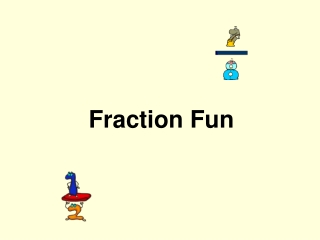DownloadDownload PresentationFraction Fun

# Fraction Fun

Télécharger la présentation## Fraction Fun

- - - - - - - - - - - - - - - - - - - - - - - - - - - E N D - - - - - - - - - - - - - - - - - - - - - - - - - - -
##### Presentation Transcript

1. Fraction Fun

2. What is a fraction A fraction names a part of an object

3. Hurry up!I have a question to ask. Are you hungry?

4. Because I ate of your pizza! It was delicious!

5. =

6. Numerator Denominator

7. Always check to make sure the fraction is reduced to lowest terms Click on the picture below http://www.kidsolr.com/math/fractions.html

8. What is an equivalent fraction? • Two fractions that stand for the same number

9. Was that simple enough? Well then go to the next page already!

10. Improper Fractions Mixed Numbers Vs.

11. What is a mixed number? The sum of a whole number and a fraction

12. & These are examples of mixed numbers

13. What is an improper fraction A fraction with a numerator greater then the denominator NUMERATOR denominator

14. & These are examples of improper fractions

15. Notice how the denominator stays the same when converting to an improper fraction to a mixed number =

16. Notice how the denominators stay the same when converting from a mixed number to an improper fraction = =

17. Were here to show you the rules! Multiplying Fractions Adding Fractions Dividing Fractions Subtracting Fractions

18. Adding Fractions Adding fractions requires a common denominator A + C = AD + CB = AD + CB B + D = BD + BD = BD

19. Subtracting Fractions Subtracting fractions requires a common denominator A - C = AD - CB = AD - CB B - D = BD - BD = BD

20. Multiplying Fractions Multiplying fractions is easy A * C = AC B * D = BD

21. Dividing Fractions Dividing fractions requires one more step A / C = A*D = AD B D = B*C BC

22. Click on the hyperlink below so you can practice adding, subtracting, multiplying, and dividing fractions! http://www.aplusmath.com/Worksheets/Fractions.htm

23. The End The End